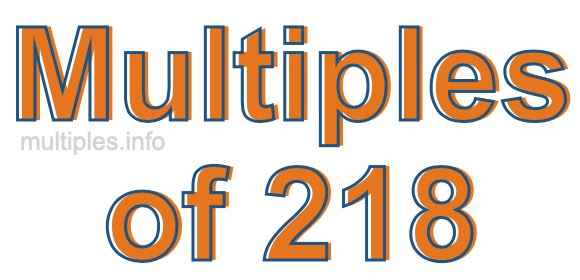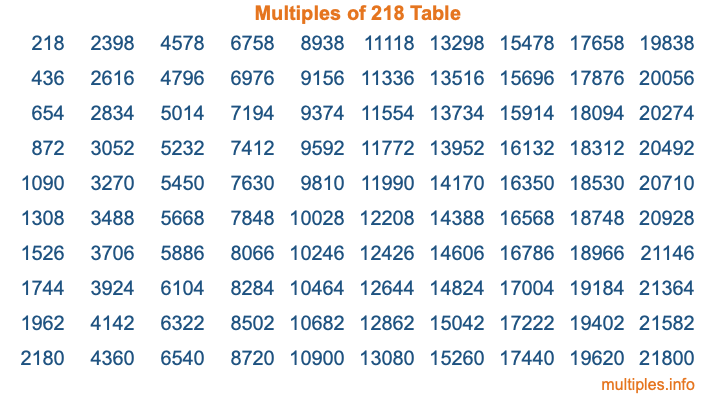Multiples of 218Welcome to the Multiples of 218 page. Here we will first teach you everything you will ever need to know about the multiples of 218, and then give you a study guide summary of everything we taught you to make sure you remember it all. Use this page to look up facts and learn information about the multiples of 218. This page will make you a multiples of two hundred eighteen expert!

Definition of Multiples of 218
Multiples of 218 are all the numbers that when divided by 218 equal an integer. Each of the multiples of 218 are called a multiple. A multiple of 218 is created by multiplying 218 by an integer.

Therefore, to create a list of multiples of 218, you start with 1 multiplied by 218, then 2 multiplied by 218, then 3 multiplied by 218, and so on for as long as you want. Thus, the list of the first five multiples of 218 is 218, 436, 654, 872, and 1090. To see a larger list of multiples of 218, see the printable image of Multiples of 218 further down on this page. We also have a category where you can choose any nth multiple of 218.

Multiples of 218 Checker
The Multiples of 218 Checker below checks to see if any number of your choice is a multiple of 218. In other words, it checks to see if there is any number (integer) that when multiplied by 218 will equal your number. To do that, we divide your number by 218. If the the quotient is an integer, then your number is a multiple of 218.

Is  a multiple of 218?

Least Common Multiple of 218 and ...
A Least Common Multiple (LCM) is the lowest multiple that two or more numbers have in common. This is also called the smallest common multiple or lowest common multiple and is useful to know when you are adding our subtracting fractions. Enter one or more numbers below (218 is already entered) to find the LCM.

Check out our LCM Calculator if you need more details about the Least Common Multiple or if you need the LCM for different numbers for adding and subtraction fractions.

nth Multiple of 218
As we stated above, 218 is the first multiple of 218, 436 is the second multiple of 218, 654 is the third multiple of 218, and so on. Enter a number below to find the nth multiple of 218.

th multiple of 218

Multiples of 218 vs Factors of 218
218 is a multiple of 218 and a factor of 218, but that is where the similarities end. All postive multiples of 218 are 218 or greater than 218. All positive factors of 218 are 218 or less than 218.

Below is the beginning list of multiples of 218 and the factors of 218 so you can compare:

Multiples of 218: 218, 436, 654, 872, 1090, etc.

Factors of 218: 1, 2, 109, 218

As you can see, the multiples of 218 are all the numbers that you can divide by 218 to get a whole number. The factors of 218, on the other hand, are all the whole numbers that you can multiply by another whole number to get 218.

It's also interesting to note that if a number (x) is a factor of 218, then 218 will also be a multiple of that number (x).

Multiples of 218 vs Divisors of 218
The divisors of 218 are all the integers that 218 can be divided by evenly. Below is a list of the divisors of 218.

Divisors of 218: 1, 2, 109, 218

The interesting thing to note here is that if you take any multiple of 218 and divide it by a divisor of 218, you will see that the quotient is an integer.

Multiples of 218 Table
Below is an image of the first 100 multiples of 218 in a table. The table is in chronological order, column by column. The first column has the first ten multiples of 218, the second column has the next ten multiples of 218, and so on.The Multiples of 218 Table is also referred to as the 218 Times Table or Times Table of 218. You are welcome to print out our table for your studies.

Negative Multiples of 218
Although not often discussed or needed in math, it is worth mentioning that you can make a list of negative multiples of 218 by multiplying 218 by -1, then by -2, then by -3, and so on, to get the following list of negative multiples of 218:

-218, -436, -654, -872, -1090, etc.

Multiples of 218 Summary
Below is a summary of important Multiples of 218 facts that we have discussed on this page. To retain the knowledge on this page, we recommend that you read through the summary and explain to yourself or a study partner why they hold true.

There are an infinite number of multiples of 218.

A multiple of 218 divided by 218 will equal a whole number.

218 divided by a factor of 218 equals a divisor of 218.

The nth multiple of 218 is n times 218.

The largest factor of 218 is equal to the first positive multiple of 218.

218 is a multiple of every factor of 218.

218 is a multiple of 218.

A multiple of 218 divided by a divisor of 218 equals an integer.

218 divided by a divisor of 218 equals a factor of 218.

Any integer times 218 will equal a multiple of 218.

Multiples of a Number
Here you can get the multiples of another number, all with the same attention to detail as we did for multiples of 218 on this page.

Multiples of
Multiples of 219
Did you find our page about multiples of two hundred eighteen educational? Do you want more knowledge? Check out the multiples of the next number on our list!